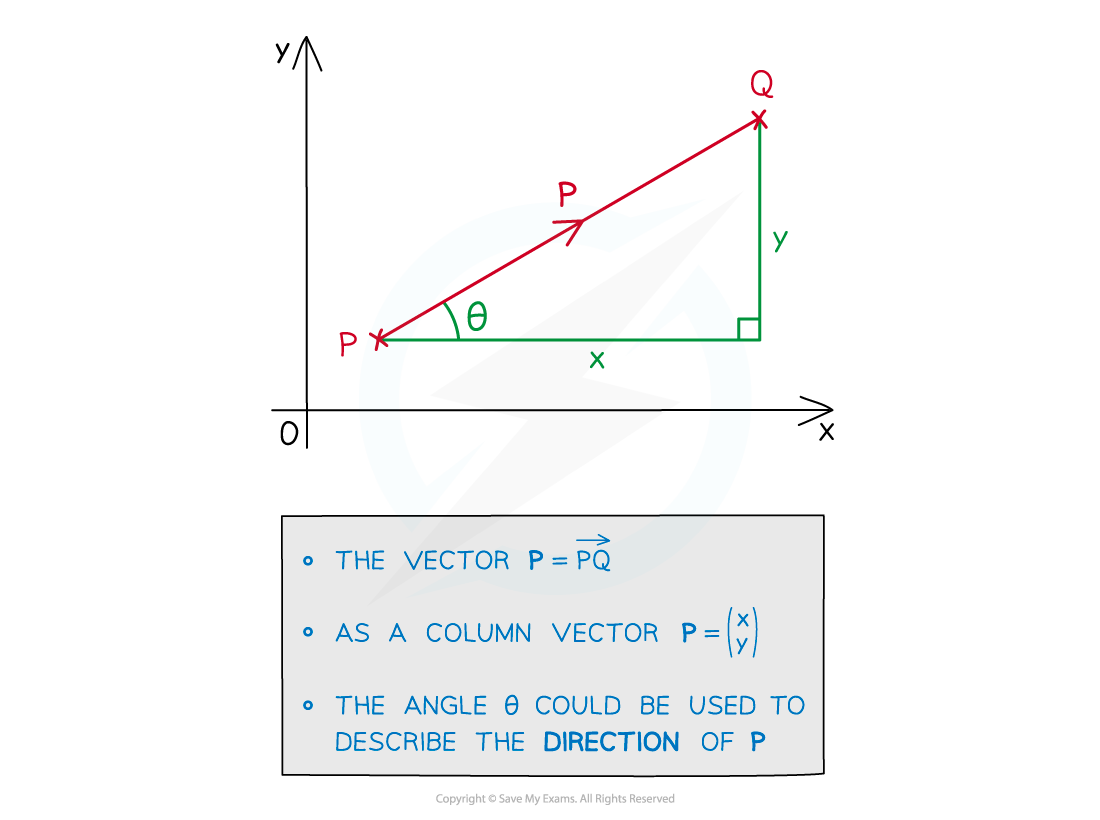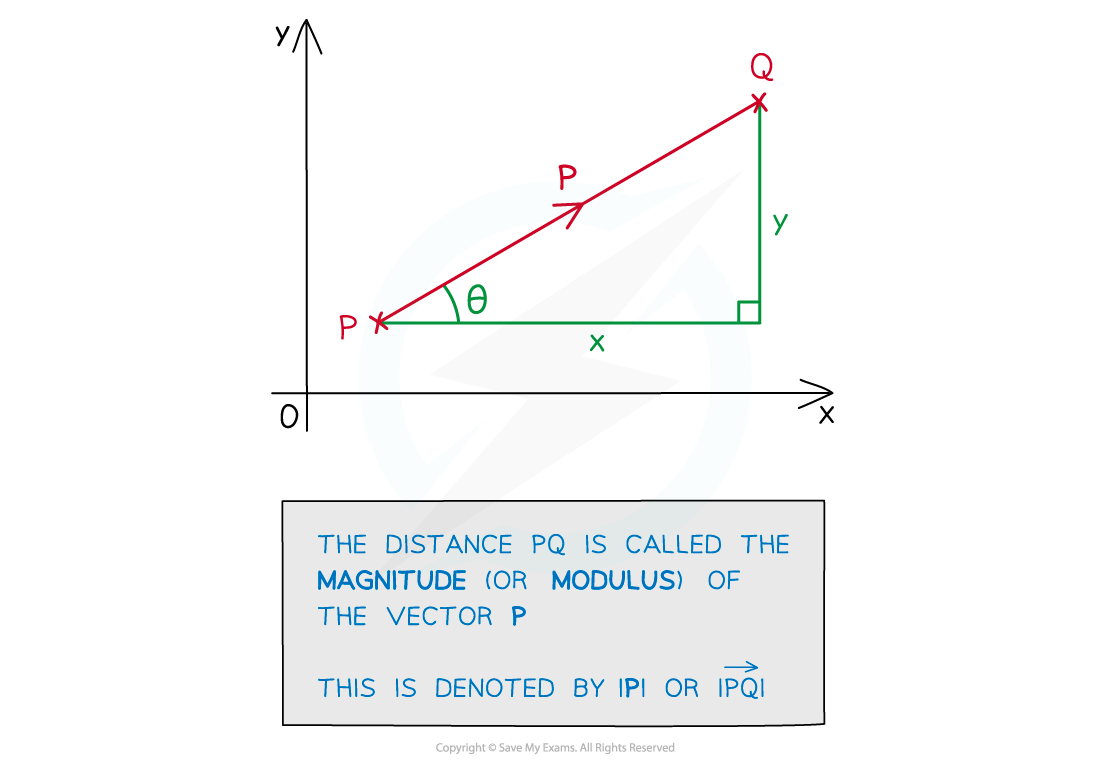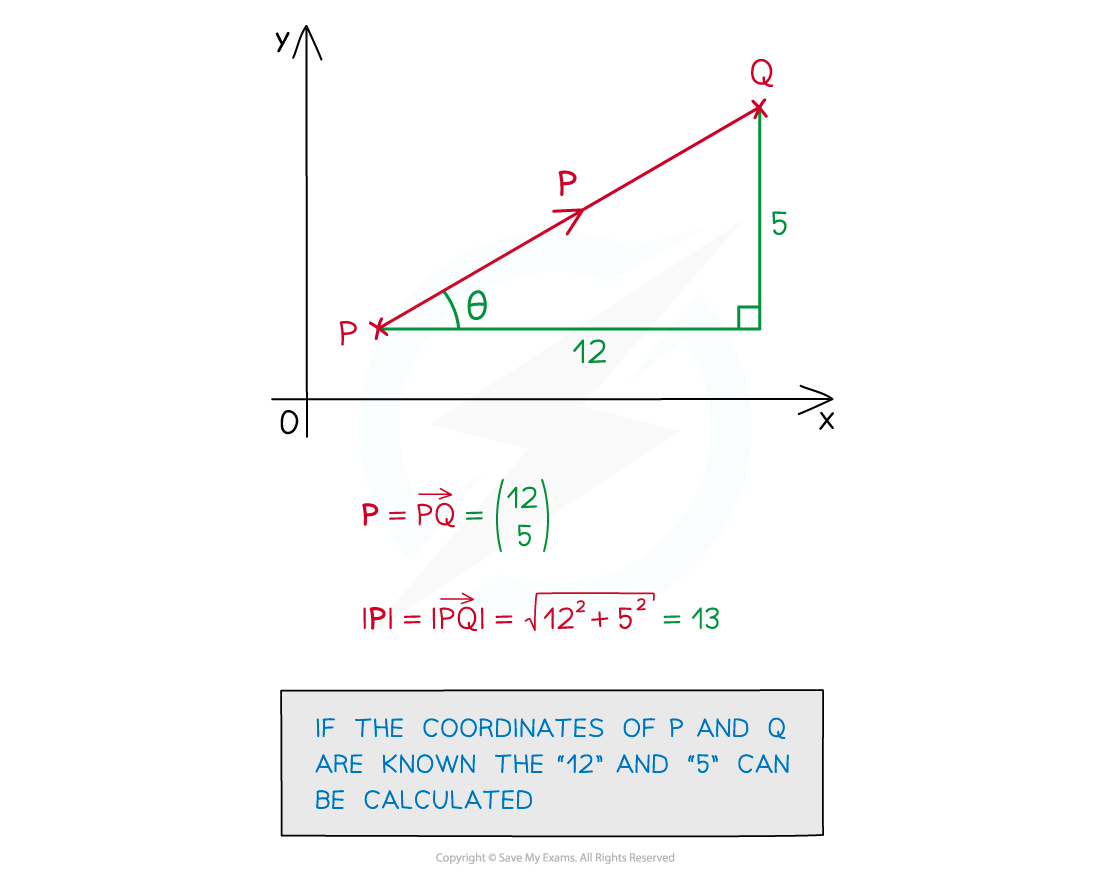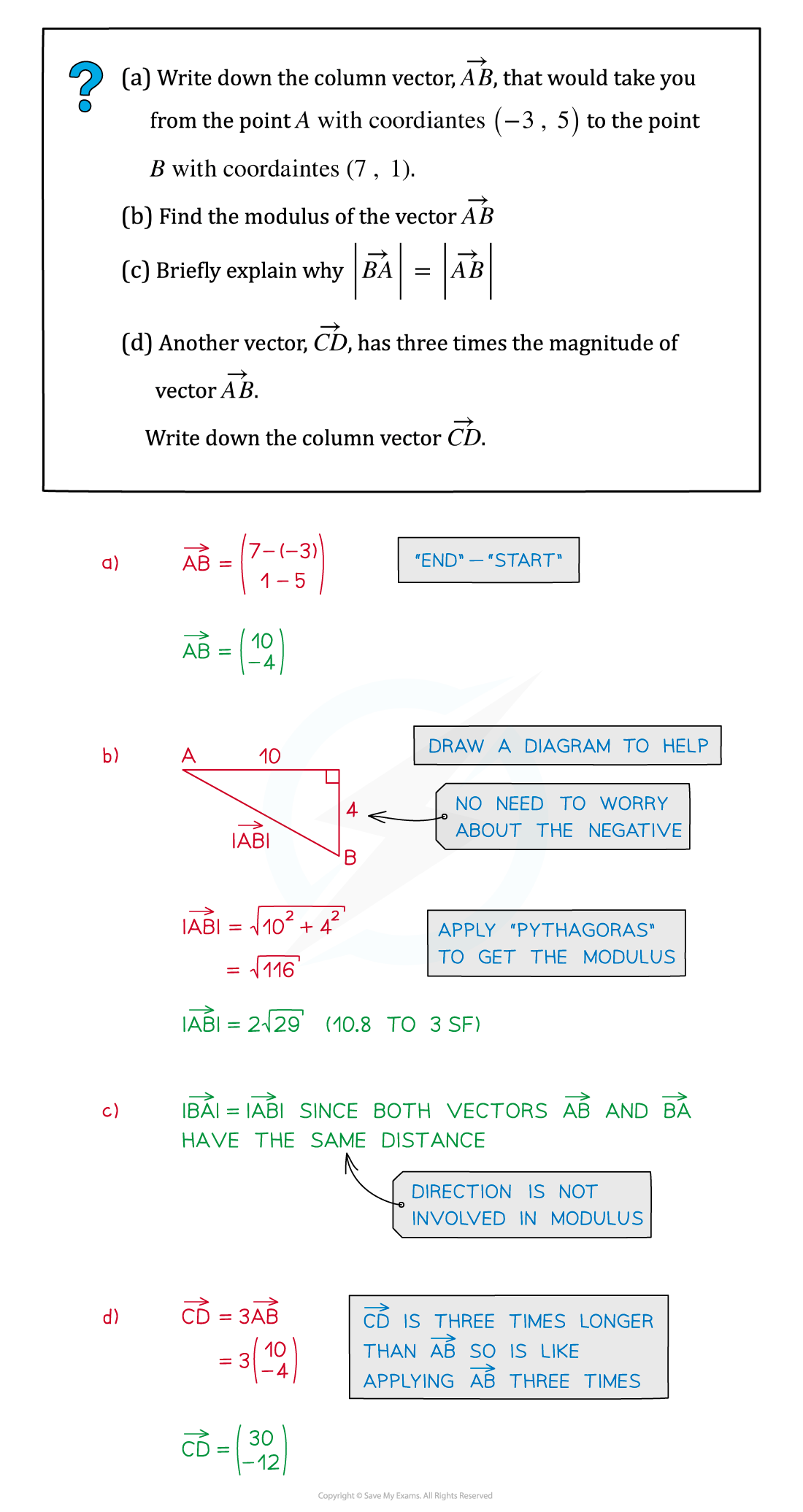# Edexcel IGCSE Maths 复习笔记 5.1.4 Vectors - Modulus

Edexcel IGCSE Maths 复习笔记 5.1.4 Vectors - Modulus

#### What is a vector?• Vectors have various uses in mathematics
• In mechanics vectors represent velocity, acceleration and forces
• At IGCSE vectors are used in geometry – eg. translation
• Ensure you are familiar with the Revision Notes Vectors – Basics

• Vectors have magnitude and direction
• These notes look at finding the magnitude, or modulus, of a vector
• Vectors are given in column vector form

#### What is the magnitude or modulus of a vector?• This depends on the use of the vector
• For velocity, magnitude would be speed
• For a force, magnitude would be the strength of the force (in Newtons)

• The words magnitude and modulus mean the same thing with vectors
• In geometry magnitude and modulus mean the distance of the vector
• This is always a positive value
• The direction of the vector is irrelevant

• Magnitude or modulus is indicated by vertical lines
• |a| would mean the magnitude of vector a

#### How do I find the magnitude or modulus of a vector

• Pythagoras’ Theorem!#### Exam Tip

Sketch a vector to help, it does not have to be to scale – you can then use this to form a right-angled triangle.

#### Worked Example